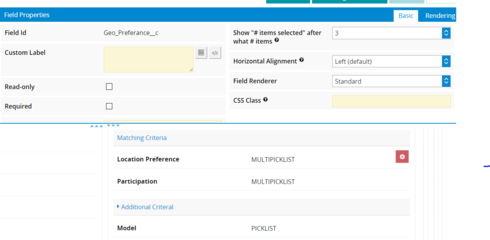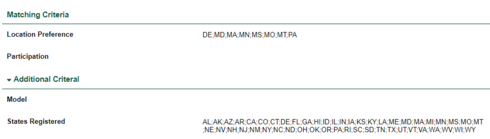# Multi select pick-list showing all values, not # items selected

Hello,

I have a couple different multi select pick list fields with all of the states as value options. I would like to show the values if there are 1, 2, or 3 selected states but then switch to show something like '# items selected' after that.

I know there is an option for this in edit mode but I would like this for view mode as well. Is there a way to do this? I would like to not have to use a snippet it I can help it but understand that I might need to.

Screen shots:`<skuidpage personalizationmode="server" showsidebar="true" useviewportmeta="true" showheader="true">    <models>        <model id="UIOnlyModel" query="true" createrowifnonefound="true" datasource="Ui-Only" processonclient="true">            <fields>                <field id="MultiselectField" displaytype="MULTIPICKLIST" label="MultiselectField" ogdisplaytype="TEXT" picklistsource="manual">                    <picklistentries>                        <entry value="One" label="One"/>                        <entry value="Two" label="Two"/>                        <entry value="Three" label="Three"/>                        <entry value="Four" label="Four"/>                        <entry value="Five" label="Five"/>                    </picklistentries>                </field>                <field id="Count" displaytype="FORMULA" label="Count" ogdisplaytype="TEXT" readonly="true" returntype="DOUBLE" precision="9" scale="0">                    <formula>customString__COUNT_SELECTED({{MultiselectField}})</formula>                </field>            </fields>            <conditions/>            <actions/>        </model>    </models>    <components>        <grid uniqueid="sk-3DdT-292">            <divisions>                <division behavior="flex" minwidth="100px" ratio="1">                    <components>                        <basicfieldeditor showheader="true" showsavecancel="false" showerrorsinline="true" model="UIOnlyModel" uniqueid="sk-3ho-Kk-479" mode="edit">                            <columns>                                <column width="100%">                                    <sections>                                        <section title="Count Multi-select Options Using Custom Formula" collapsible="no">                                            <fields>                                                <field uniqueid="sk-3DdT-221" id="MultiselectField" selectedlist="3">                                                    <label>FIeld {{Count}} selected</label>                                                    <renderconditions logictype="and" onhidedatabehavior="keep"/>                                                    <enableconditions/>                                                </field>                                                <field uniqueid="sk-3DNF-1172" id="Count"/>                                            </fields>                                        </section>                                    </sections>                                </column>                            </columns>                        </basicfieldeditor>                    </components>                </division>                <division behavior="flex" verticalalign="top" minwidth="100px" ratio="1">                    <components>                        <basicfieldeditor showheader="true" showsavecancel="false" showerrorsinline="true" model="UIOnlyModel" uniqueid="sk-3DdT-272" mode="edit">                            <columns>                                <column width="100%">                                    <sections>                                        <section title="Show/Hide using Count to Render Field" collapsible="no">                                            <fields>                                                <field uniqueid="sk-3DdT-273" id="MultiselectField" selectedlist="3">                                                    <renderconditions logictype="and" onhidedatabehavior="keep">                                                        <rendercondition type="fieldvalue" operator="lte" enclosevalueinquotes="false" fieldmodel="UIOnlyModel" sourcetype="fieldvalue" field="Count" value="3"/>                                                    </renderconditions>                                                    <enableconditions/>                                                </field>                                                <field uniqueid="sk-3DdT-275" id="Count">                                                    <label>MultiselectField (count)</label>                                                    <renderconditions logictype="and" onhidedatabehavior="keep">                                                        <rendercondition type="fieldvalue" operator="gte" enclosevalueinquotes="false" fieldmodel="UIOnlyModel" sourcetype="fieldvalue" field="Count" value="4"/>                                                    </renderconditions>                                                    <enableconditions/>                                                </field>                                            </fields>                                        </section>                                    </sections>                                </column>                            </columns>                        </basicfieldeditor>                    </components>                </division>            </divisions>            <styles>                <styleitem type="background" bgtype="none"/>            </styles>        </grid>    </components>    <resources>        <labels/>        <javascript>            <jsitem location="inline" name="customFormulas" cachelocation="false" url="">skuid.formula.Formula (  'COUNT_SELECTED',  function (multiselectfield) {      var num;      if (multiselectfield===null) {        num = 0;            } else {        num =  (String(multiselectfield).match(/;/g) || []).length + 1;      }    return num;  },{    namespace: 'customString',    numArgs : 1,    returnType : 'number'  });</jsitem>        </javascript>        <css/>        <actionsequences uniqueid="sk-3honBl-265"/>    </resources>    <styles>        <styleitem type="background" bgtype="none"/>    </styles></skuidpage>`